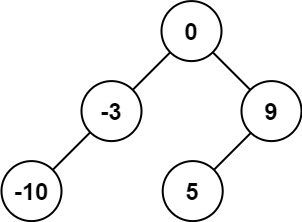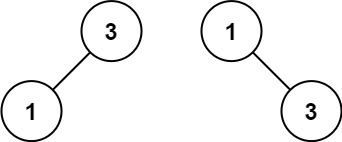0108. 将有序数组转换为二叉搜索树

# 0108. 将有序数组转换为二叉搜索树#

• 标签：树、深度优先搜索
• 难度：简单

## 题目大意 #

• $1 \le nums.length \le 10^4$。
• $-10^4 \le nums[i] \le 10^4$。
• nums 按严格递增顺序排列。

• 示例 1：1 2 3  输入：nums = [-10,-3,0,5,9] 输出：[0,-3,9,-10,null,5] 解释：[0,-10,5,null,-3,null,9] 也将被视为正确答案 
• 示例 2：1 2 3  输入：nums = [1,3] 输出：[3,1] 解释：[1,null,3] 和 [3,1] 都是高度平衡二叉搜索树。 

## 解题思路 #

### 思路 1：代码 #

  1 2 3 4 5 6 7 8 9 10 11  class Solution: def sortedArrayToBST(self, nums: List[int]) -> Optional[TreeNode]: def build(left, right): if left > right: return mid = left + (right - left) // 2 root = TreeNode(nums[mid]) root.left = build(left, mid - 1) root.right = build(mid + 1, right) return root return build(0, len(nums) - 1) 

### 思路 1：复杂度分析 #

• 时间复杂度：$O(n)$。其中 $n$ 是数组的长度。
• 空间复杂度：$O(n)$。